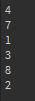# Using table.sort() on string indexes doesn't work

``````local tab = {}
tab['hello'] = 4
tab['bing'] = 8
tab['yay'] = 7
tab['heasf'] = 3
tab['yshdfy'] = 1

table.sort(tab)

for i,v in pairs(tab) do
print(tab[i])
end
``````

Output:If table.sort doesn’t support string indexes, is there another way I can use to sort these string indexes out from big to small?

You can’t sort keys, only indexes.

From the Developer Hub

Sorts elements of array t in a given order, from t to t[#t]. If comp is given, then it must be a function that receives two elements and returns true when the first element must come before the second in the final order (so that not comp(t[i+1],t[i]) will be true after the sort). If comp is not given, then the standard Lua operator `<` is used instead.

It only works with arrays, not dictionaries.

Having a long look at this, there isn’t really a way to solve this issue easily. What’s your specific use case for `table.sort` that requires a dictionary?

To sort the players’ scores

``````local plrs = {}
plrs['coolboy123'] = 216
plrs['funfungamers'] = 683
plrs['asdasdasd'] = 120
``````

Unfortunately, there’s no easy way around this. Dictionaries, unlike arrays, cannot have an order.

# Solution 1 Convert to Array

You’ll need to convert it to arrays like this.

``````local players = {
{'coolboy123', 216},
{'funfungamers', 683},
{'asdasdasd', 120}
}
``````

And then sort the table with the following code:

``````table.sort(players, function(a, b)
return a > b
end)
``````

Our table is now:

``````funfungamers 683
coolboy123 216
asdasdasd 120
``````

# Solution 2 spairs

Credit to this StackOverflow post

Here’s a modified version of pairs- called spairs
(This is in a modulescript)

``````return function (t, order)
-- collect the keys
local keys = {}
for k in pairs(t) do keys[#keys+1] = k end

-- if order function given, sort by it by passing the table and keys a, b,
-- otherwise just sort the keys
if order then
table.sort(keys, function(a,b) return order(t, a, b) end)
else
table.sort(keys)
end

-- return the iterator function
local i = 0
return function()
i = i + 1
if keys[i] then
return keys[i], t[keys[i]]
end
end
end
``````

Here’s an example use:

``````local spairs = require(script.spairs)

local plrs = {}
plrs['coolboy123'] = 216
plrs['funfungamers'] = 683
plrs['asdasdasd'] = 120

local function order(t, a, b)
return t[b] < t[a]
end

for i,v in spairs(plrs, order) do
print(i, v)
end
``````

Our output is as expected.

``````funfungamers 683
coolboy123 216
asdasdasd 120
``````
6 Likes